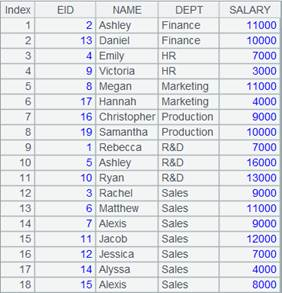• 集算器
教程
函数参考
用户参考
外部库使用指南
数据文件工具用户参考
DQL教程
集群管理器使用说明
SPL命令执行器用户参考
• 润乾报表
教程
填报教程
分析教程
报表中心教程
用户参考
程序员参考
高级指南
通用查询
DQL部署集成手册
• 易明建模
用户参考
json参数说明
• 润乾官网

# group()

## A.group(x i ,…)

xi,…做等值分组

A.group(xi,…)

 @o 只和相邻对比，相当于归并，结果集不再排序 @1 取每个分组的第一条记录，组成排列后返回（注意是数字1，不是字母l），可以与@v配合使用 @n x取值为分组序号，可直接定位；与@o互斥；将x<1的数据组丢弃 @u 结果集不再按x排序；与@o/@n互斥 @i x是布尔表达式，如果x的结果为true则开始新的一组。相当于A.group@o(a+=if(x,1,0))中a=0且只有一个x。 @0 将x的计算结果为空的组丢弃，当只有一个x时可以用该选项，与@n一起使用时，也将空组丢弃 @s 分组后序列/排列和列，相当于A.group(xi,…).conj()，可以与@v配合使用 @p 返回组成员在A中位置构成的数列的序列 @h 用于分段有序的数据，可提高分组效率 @v A为纯序表时，返回成纯序表构成的集合

 A 序列 xi 分组表达式

Ø  对数列分组

 A 1 [6,9,12,15,16,5,1,7,8] [[6,12,16,8],[ 9,15,5,1,7]]数列被分为2组，一组成员除以2余数为0，另一组成员除以2余数为1 2 =A1.group(~%2) 3 =A1.group(~%2,~%3) [[6,12],,,[9,15],[1,7],]按照多个表达式分组。 4 =[6,9,16,5,1,7,8].group@s(~%2)按照奇偶数分组后和列 5 =A1.group((#-1)\3) 对序列A1分组，每3个一组Ø  对分组结果重复利用

 A 1 =demo.query("select NAME,BIRTHDAY,GENDER from EMPLOYEE") 2 =A1.group(GENDER) A1序表根据GENDER分组：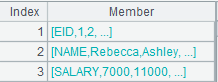点开分组后每个成员就是一个序列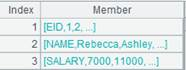3 =A2.new(GENDER:Gender,~.count():Number)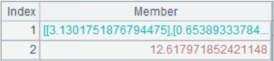对分组后的成员统计 4 =A2.new(GENDER:Gender,~.avg(age(BIRTHDAY)):Average)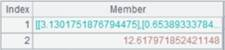对分组结果重复利用，再次进行不同的统计

Ø  多种分组方式

 A 1 =demo.query("select NAME,GENDER,DEPT,BIRTHDAY from EMPLOYEE") 2 =A1.group(GENDER,DEPT) 多字段分组 3 =A1.group@o(GENDER) 不排序，相邻的记录比较，相同的记录归为一组。不相邻但相同的记录可能变成两组，因此会出现重复的组，返回序列集4 =A1.group@1(GENDER)返回每组第一条记录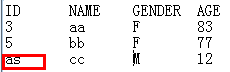5 =A1.group@n(if(GENDER=="F",1,2)) x取值为分组序号，可直接定位 [[Rebecca,Ashley,Rachel,…],[Matthew,Ryan,Jacob,…]] 6 =A1.group@u(GENDER,DEPT) 结果集不按分组字段排序 7 =A1.group@i(GENDER=="F")遇到GENDER=="F"则开始新的分组 8 =A1.group@p(GENDER,DEPT)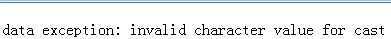返回按照字段GENDER,DEPT分组后，记录在原序表中的位置构成的序列 9 =file("D:\\Salesman.txt").import@t()10 =A9.group@0(Gender)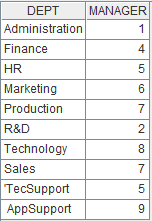按照字段Gender分组，为空的组丢弃不显示 11 =file("D:/emp10.txt").import@t() 数据文件emp10.txt中，每10条数据根据DEPT进行了一次排序12 =A11.group@h(DEPT) A11是以DEPT分段有序的数据，使用@h选项提高分组效率13 =A1.group@n(if(DEPT=="HR":1,DEPT=="Sales":2;0)) 使用@n选项，函数参数值小于1的数据丢弃，即将DEPT不为HR也不是Sales的数据组丢弃Ø  A为序表

 A 1 =demo.query@v("select * from EMPLOYEE order by GENDER,DEPT ") 返回纯序表 2 =A1.group@v(GENDER,DEPT) 使用@v选项，返回纯序表构成的集合

## A.group(x:F,...;y:G,…)

A.group(x:F,...;y:G,…)

 @o 只和相邻对比，相当于归并，结果集不再排序 @n x取值为分组序号，可直接定位；与@o互斥 @u 结果集不再按x排序；与@o/@n互斥 @i x是布尔表达式，如果x的结果为true则开始新的一组。此时只有一个x。 @0 将x的计算结果为空的组丢弃 @h 用于分段有序的数据，可提高分组效率 @t 当A的返回值为空，则返回一个保留数据结构的空序表 @s 以累计方式计算

 A 序列 x 分组表达式，x:F省略则不分组针对全集聚合，此时分号；不可省略 F 结果序表的字段名 G 结果序表中的汇总字段名 y 聚合表达式，用~引用组

 A 1 =demo.query("select * from SCORES") 2 =A1.group(STUDENTID:StudentID;~.sum(SCORE):TotalScore)3 =A1.group@o(STUDENTID:StudentID;~.sum(SCORE):TotalScore)只和相邻的对比归并，结果集不排序 4 =demo.query("select * from STOCKRECORDS where STOCKID<'002242'") 5 =A4.group@n(if(STOCKID=="000062",1,2):StockID;~.sum(CLOSING):TotalPrice)x取值为分组序号 6 =A1.group(;~.sum(SCORE):TotalScore)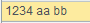省略x:F求所有学生的总成绩 7 =demo.query("select * from EMPLOYEE") 8 =A7.group@u(STATE:State;~.count(STATE):TotalScore) 结果集不按分组字段排序 9 =A7.group@i((GENDER=="F"):IsF;~.count():Number)遇到GENDER=="F"则开始新的分组 10 =file("D:\\Salesman.txt").import@t()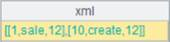11 =A10.group@0(Gender:Gender;~.sum(Age):Total)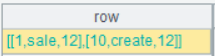Gender为空的组丢弃 12 =file("D:/emp10.txt").import@t() 数据文件emp10.txt中，每10条数据根据DEPT进行了一次排序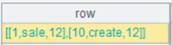13 =A12.group@h(DEPT:dept;~.sum(SALARY):bouns) A12是以DEPT分段有序的数据，使用@h选项提高分组效率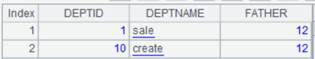14 =demo.query("select * from EMPLOYEE where EID > 500") 返回空序表 15 =A14.group@t(STATE:State;~.count(STATE):TotalScore) 返回保留数据结构的空序表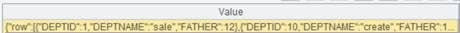16 =demo.query("select * from employee").group@s(DEPT;sum(SALARY):TotleSalary) 以累计方式计算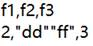## ch.group()

ch.group(x)

 @i x是布尔表达式，如果x的结果为true则开始新的一组。此时只有一个x。

 ch 管道 x 分组表达式，多个字段/表达式组合分组时，每个分组表达式用逗号隔开

 A 1 =demo.cursor("select EID,NAME,GENDER,DEPT,SALARY from EMPLOYEE") 2 =channel() 创建管道 3 =channel() 创建管道 4 =A2.group(GENDER,DEPT) 按照GENDER,DEPT分组 5 =A2.fetch() A2管道附加结果集函数ch.fetch()，保留管道当前数据 6 =A3.group@i(GENDER=="F") 遇到GENDER=="F"则开始新的分组 7 =A3.fetch() A3管道附加结果集函数ch.fetch() 8 =A1.push(A2,A3) 将游标A1中的数据推送到管道A2和A3，此时数据不会立即被推送到管道。 9 =A1.skip() A1执行skip()时程序才会执行管道中的计算，游标中的数据才真正被推送到管道。 10 =A2.result()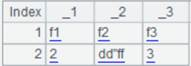11 =A3.result()## cs.group()

cs.group(x,…)

 @i x是布尔表达式，如果x的结果为true则开始新的一组。此时只有一个x。 @1 取每组的第一条记录组成排列返回到原游标（注意是数字1，不是字母l） @v cs是纯序表游标时，每组子集将复制成新的纯序表

 cs 游标/多路游标记录 x 分组表达式

 A 1 =demo.cursor("select * from EMPLOYEE").sortx(GENDER,DEPT) 返回游标中的数据对GENDER、DEPT有序 2 =A1.group(GENDER,DEPT) 相邻记录中GENDER与DEPT字段值都相同时，记录分到同一组中，结果返回原游标 3 =demo.cursor("select * from EMPLOYEE") 4 =A3.group@i(GENDER=="F").fetch() 遇到GENDER=="F"则开始新的分组5 =demo.cursor("select * from EMPLOYEE order by GENDER") 6 =A5.group@1 (GENDER).fetch() 返回每组第一条记录组成的排列cs为纯序表游标

 A 1 =demo.cursor@v("select * from EMPLOYEE order by GENDER,DEPT ") 返回纯序表游标 2 =A1.group@v(GENDER,DEPT) 使用@v选项，每组子集将复制成新的纯序表 3 =A2.fetch()

## cs.group()

cs.group(x,…)

 cs 集群游标记录 x 分组表达式，多个字段/表达式组合分组时，每个分组表达式用逗号隔开

 A 1 =file("employees.ctx","192.168.0.111:8281") employees.ctx文件对DEPT有序，数据内容如下：2 =A1.open() 打开集群组表 3 =A2.cursor() 返回集群游标 4 =A3.group(DEPT) 将集群游标记录根据DEPT分组，结果返回原集群游标 5 =A4.fetch()## cs.group(x:F,…;y:G,…)

cs游标做相邻分组聚合，返回原游

 cs 游标记录 x 分组表达式 F 字段名 G 汇总字段名 y 聚合表达式

 @s 用累积方式聚合 @q(x:F,…;x’:F’,…;…) cs已对x,…有序，仅后面的字段需要排序时可用该选项，可以内存排序。 @sq(x:F,…;x’:F’,…;…) 没有y:G参数时仅排序，不分组；有y:G参数时按累计方式聚合。该用法中@s必须与@q配合使用。 @e 返回y的结果组成的序表。其中x为A的字段名，y是A的函数，y的计算结果要求是A的一条记录，当y是聚合函数时仅支持maxp/minp/top@1。 组合使用@sev选项时，返回结果为纯序表

 A 1 =demo.cursor("select * from SCORES  where STUDENTID <5  order by  STUDENTID ") 按照SCORE排序 2 =A1.group(STUDENTID:StudentID;~.sum(SCORE):TotalScore) 返回原游标cs 3 =A2.fetch()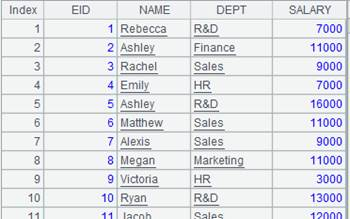4 =demo.cursor("select * from SCORES  where STUDENTID <5  order by  STUDENTID ") 5 =A4.group@s(STUDENTID:StudentID;sum(SCORE):TotalScore) 用累积方式聚合 6 =A5.fetch()7 =demo.cursor("select * from EMPLOYEE order by DEPT ") 查询EMPLOYEE表中的数据，根据DEPT排序，结果返回游标 8 =A7.group@q(DEPT;GENDER).fetch()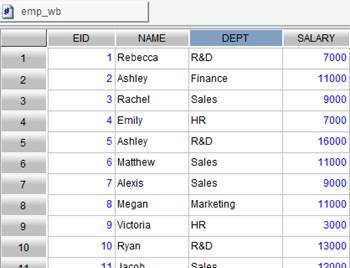A7中返回的游标已经对DEPT排序，所以分组时仅对GENDER排序 9 =demo.cursor("select * from EMPLOYEE order by DEPT ") 10 =A9.group@qs(DEPT:DEPT;GENDER:GENDER).fetch() 仅排序，不分组11 =demo.cursor("select * from EMPLOYEE order by DEPT ") 12 =A11.group@qs(DEPT:DEPT;GENDER:GENDER;count(GENDER):count).fetch()按累计方式聚合

 A 1 =demo.cursor("select * from EMPLOYEE order by DEPT ") 2 =A1.group@e(DEPT;~.minp(SALARY)).fetch()返回由minp(SALARY)结果记录构成的游标

## cs.group(x:F,…;y:G,…)

cs.group(x:F,...;y:G,…)

 cs 集群游标 x 分组表达式 F 字段名 G 汇总字段名 y 聚合表达式

 A 1 =file("employees.ctx","192.168.0.111:8281") employees.ctx文件对DEPT有序，数据内容如下：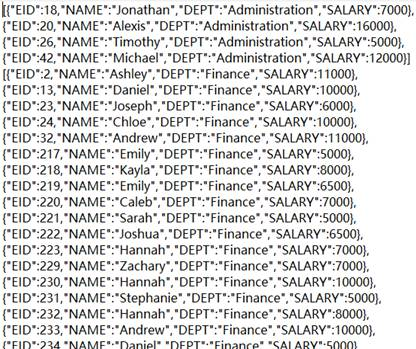2 =A1.open() 打开集群组表 3 =A2.cursor() 返回集群游标 4 =A3.group(DEPT:dept;count(NAME):count) 根据DEPT相邻分组，然后聚合，结果返回原集群游标 5 =A4.fetch()## T. group ()

T.group(xi,…)

 T 虚表 xi 分组表达式，多个字段/表达式组合分组时，每个分组表达式用逗号隔开

 @i x是布尔表达式，如果x的结果为true则开始新的一组。此时只有一个x @1 取每一个分组的第一条记录组成排列后返回（注意是数字1，不是字母l） @t 返回成序表，可以直接建索引，缺省是排序 @v 组表第一次加载时用列式，提升性能

 A 1 =create(file).record(["D:/file/pseudo/empT.ctx"]) 2 =pseudo(A1) 生成虚表对象 3 =A2.group(GENDER,DEPT) 相邻记录中GENDER与DEPT字段值皆相同时，记录分到同一组中，结果返回新虚表 4 =A3.import() 取出虚表数据5 =A2.group@i(GENDER=="F") 遇到GENDER=="F"则开始新的分组 6 =A5.import() 取出虚表数据7 =A2.group@1t(GENDER) 返回每组第一条记录

## T.group(x:F,…;y:G,…)

T.group(x:F,...;y:G,…)

 T 虚表 x 分组表达式 F 字段名 G 汇总字段名 y 聚合表达式

 @s 用累积方式聚合 @q(x:F,…;x’:F’,…;…) T已对x,…有序，仅后面的字段需要排序时可用该选项，可以内存排序。 @sq(x:F,…;x’:F’,…;…) 没有y:G参数时仅排序，不分组；有y:G参数时按累计方式聚合。该用法中@s必须与@q配合使用。 @e 返回y的结果组成的虚表。其中x为T的字段名，y是T的函数，y的计算结果要求是T的一条记录，当y是聚合函数时仅支持maxp/minp/top@1。

 A 1 =create(file).record(["scores-g.ctx"]) scores-g.ctx 为对STUDENTID有序的组表文件，内容如下：2 =pseudo(A1) 由A1组表生成虚表 3 =A2.group(STUDENTID:StudentID;~.sum(SCORE):TotalScore) 将虚表A2根据STUDENTID字段分组，并计算每组的SCORE的总值 4 =A3.import() A3返回的虚表内容如下：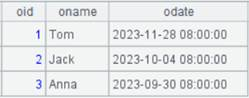A 1 =create(file).record(["emp-g.ctx"]) emp-g.ctx为对DEPT有序的组表文件，内容如下：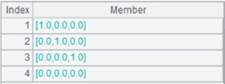2 =pseudo(A1) 由A1组表产生虚表 3 =A2.group@q(DEPT;GENDER) 虚表已经对DEPT有序，所以分组时仅对GENDER排序，返回虚表内容如下：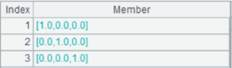4 =A2.group@qs(DEPT:DEPT;GENDER:GENDER) 没有y:G参数时仅排序，不分组，返回虚表内容如下：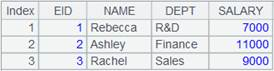5 =A2.group@qs(DEPT:DEPT;GENDER:GENDER;count(GENDER):count) 有y:G参数，按累计方式聚合，返回虚表内容如下：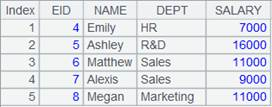A 1 =create(file).record(["emp-g.ctx"]) 组表emp-g.ctx中的内容如下：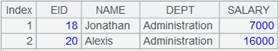2 =pseudo(A1) 由A1组表产生虚表 3 =A2.group@e(DEPT;~.minp(SALARY)) 使用@e选项，返回由minp(SALARY)结果记录构成的虚表